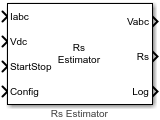# ACIM Rs Estimator

Estimate stator resistance (Rs) of AC induction motor (ACIM)

Since R2023a

•Libraries:
Motor Control Blockset / Parameter Estimation / ACIM Parameter Estimation

## Description

The ACIM Rs Estimator block computes the stator resistance of an induction motor using the phase currents, DC voltage across the motor, and the test configuration elements.

The block injects a voltage across the motor phases and measures the current response to estimate the motor resistance.

## Ports

### Input

expand all

The three-phase current response of the motor measured by the current sensors.

Note

Providing phase-c current input is optional. The block functionality is not impacted if you do not provide the phase-c current input.

Data Types: `single`

DC bus voltage that you provide to the inverter.

Data Types: `single`

Signal that either starts or stops Rs estimation:

• `1` (rising edge of a pulse) — This signal initiates the Rs estimation process.

• `0` (falling edge of a pulse) — This signal immediately stops the Rs estimation process. If you interrupt the estimation process abruptly before completion, the block does not compute or output the Rs value.

Data Types: `Boolean`

This table summarizes the expected configuration elements in the bus signal input.

CategoryConfiguration elementUnits

Elements for motor and inverter hardware

Number of pole pairs

-

Rated frequency

Hz

Nominal motor current

A

Nominal voltage

V

Maximum measurable inverter current

A

Board resistance

ohm

Current sense gain

Voltage sense gain

Elements for estimating magnetizing current Id0

Measurement time

seconds

Open-loop ramp time

seconds

Elements for estimating motor resistance (Rs)

Estimation time

seconds

Vd reference voltage 1

PU voltage

Vd reference voltage 2

PU voltage

Elements for estimating rotor resistance (Rr), magnetizing inductance (Lm), stator leakage inductance (Lls), and rotor leakage inductance (Llr)

Frequency 1 as a ratio of rated frequency

PU frequency

Frequency 2 as a ratio of rated frequency

PU frequency

High frequency for Lm

Hz

Start voltage for voltage sweep

PU voltage

Voltage step for voltage sweep

PU voltage

High-frequency injection voltage

PU voltage

Elements for estimating motor inertia (J) and friction constant (B)

Closed-loop speed duration

seconds

Closed-loop speed

RPM

Iq Reference current for FOC

PU current

Proportional gain

-

Integral gain

-

Lower speed limit for inertia estimation

RPM

-

Parameter estimation sample time

seconds

You can connect this input port to the `Config` output port of the ACIM Parameter Estimation Configurator block.

Data Types: `single`

### Output

expand all

Stator reference voltage components along the a, b, and c axes of the abc reference frame.

Data Types: `single`

Estimated stator resistance (in ohms) of the induction motor.

Data Types: `single`

Debug signals that the block provides for examination. The bus includes these debug signals.

Debug signalDescriptionUnits

Id

D-axis current

Amperes

Vd

D-axis voltage

Volts

status

`0`Did not complete Rs estimation

-

`1`Rs estimation completed successfully

-

Data Types: `single`

## Version History

Introduced in R2023a